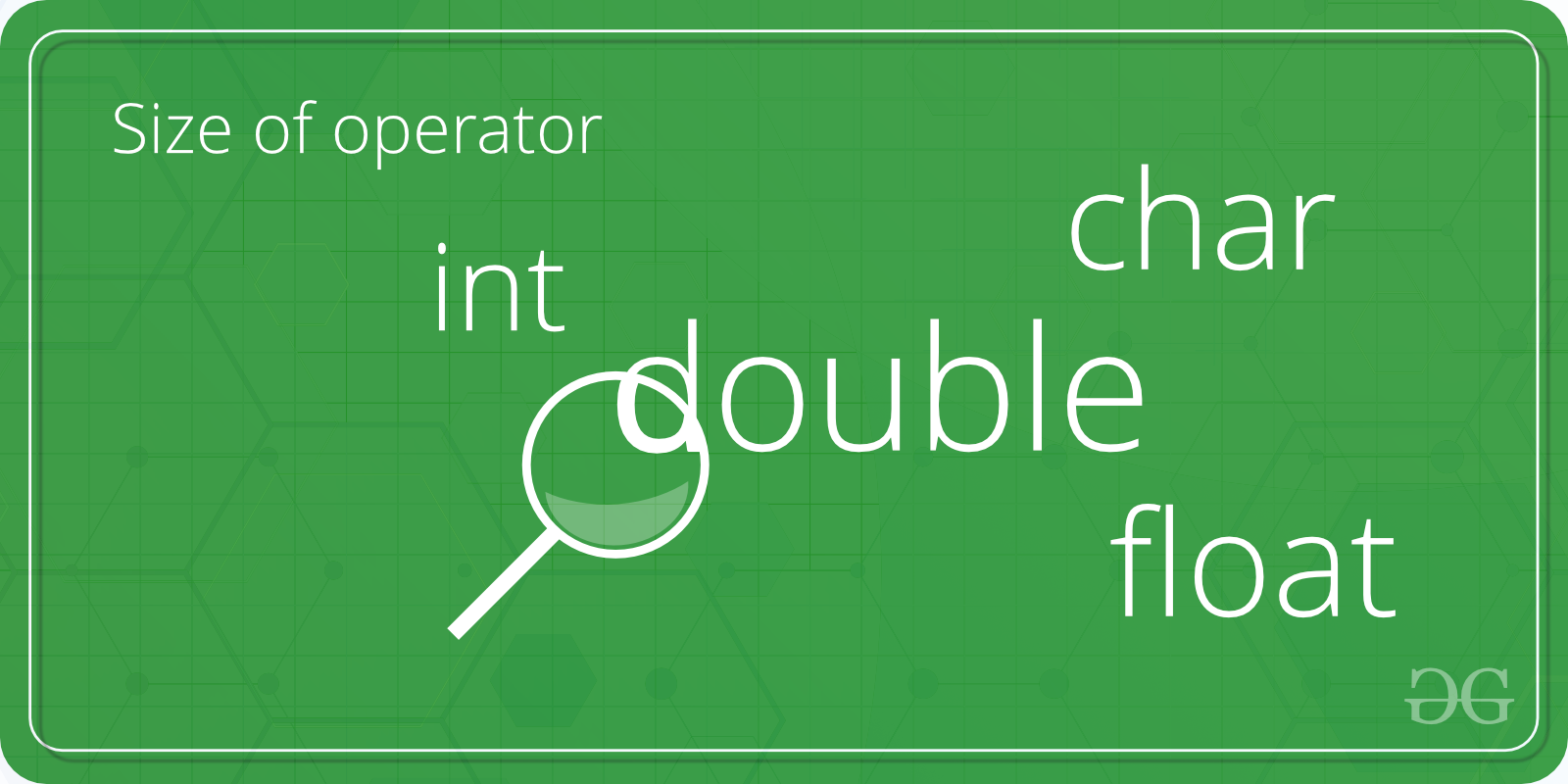Open in App
Not now

# C++ Program to Find the Size of int, float, double and char

• Difficulty Level : Basic
• Last Updated : 26 Feb, 2023

Given four types of variables, namely int, char, float and double, the task is to write a program in C++ to find the size of these four types of variables.Examples:

```Input: int
Output: Size of int = 4

Input: double
Output: Size of double = 8```

Here is a list of all the data types with its size, range and the access specifiers:

## Recommended: Please try your approach on {IDE} first, before moving on to the solution.

To find the size of the four variables:

1. The four types of variables are defined in integerType, floatType, doubleType and charType.
2. The size of the variables is calculated using the sizeof() operator.

Below is the C++ program to find the size of int, char, float and double data types:

## C++

 `// C++ program to find the size of int, char,``// float and double data types``#include ``using` `namespace` `std;` `int` `main()``{``    ``int` `integerType;``    ``char` `charType;``    ``float` `floatType;``    ``double` `doubleType;` `    ``// Calculate and Print``    ``// the size of integer type``    ``cout << ``"Size of int is: "` `<<``    ``sizeof``(integerType) <<``"\n"``;` `    ``// Calculate and Print``    ``// the size of doubleType``    ``cout << ``"Size of char is: "` `<<``    ``sizeof``(charType) <<``"\n"``;``    ` `    ``// Calculate and Print``    ``// the size of charType``    ``cout << ``"Size of float is: "` `<<``    ``sizeof``(floatType) <<``"\n"``;` `    ``// Calculate and Print``    ``// the size of floatType``    ``cout << ``"Size of double is: "` `<<``    ``sizeof``(doubleType) <<``"\n"``;` `    ``return` `0;``}`

Output:

```Size of int is: 4
Size of char is: 1
Size of float is: 4
Size of double is: 8```

Time complexity: O(1)

Auxiliary space: O(1)

As constant extra space is used.

My Personal Notes arrow_drop_up